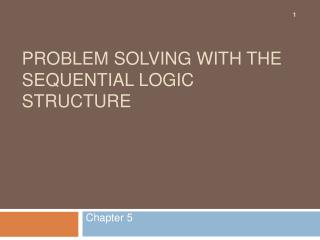Download PresentationProblem Solving with the Sequential Logic Structure

# Problem Solving with the Sequential Logic Structure - PowerPoint PPT Presentation

Problem Solving with the Sequential Logic Structure. Chapter 5. Review. Recall that the: Algorithm: set of instructions telling the computer how to process a module in a solution. A flowchart: visual illustration of an algorithm. In this chapter you will learn how to:I am the owner, or an agent authorized to act on behalf of the owner, of the copyrighted work described.
Download Presentation## Problem Solving with the Sequential Logic Structure

Download Policy: Content on the Website is provided to you AS IS for your information and personal use and may not be sold / licensed / shared on other websites without getting consent from its author.While downloading, if for some reason you are not able to download a presentation, the publisher may have deleted the file from their server.

- - - - - - - - - - - - - - - - - - - - - - - - - - E N D - - - - - - - - - - - - - - - - - - - - - - - - - -
Presentation Transcript
1. Review • Recall that the: • Algorithm: set of instructions telling the computer how to process a module in a solution. • A flowchart: visual illustration of an algorithm. • In this chapter you will learn how to: • Write an algorithm for each module in a program. • Draw a flowchart to accompany in each algorithm.

2. Algorithm Instructions and Flowchart Symbols

3. Algorithm Instructions and Flowchart Symbols

4. Exit is used to end a subordinate module if there is no return value . • Return is used when the module is to be processed within an expression . average = sum ( num1 , num2) / 2

5. Sequential Logic Structure • The most commonly used and the simplest logic structure . • all problem use the sequential structure . • most problem problems use it in conjunction with one or more of the other logic structure .

6. Sequential Logic Structure • The form of the algorithm looks like this : module name ( list of parameters) 1. instruction 2. instruction 3. ….. … x x.End,Exit,or Return (variable )

7. Flowchart Diagram for the Sequential Structure

8. Example: The algorithm and flowchart to enter a name and age into computer and print it on the screen. ( ) Notice that the algorithm instructions are numbered starting with the first instruction after the name of module

9. solution development • The problem analysis chart. • The interactivity chart . • The IPO chart . • The coupling diagram and the data dictionary . • The algorithms . • The flowcharts . • The seventh step in solving the problem is to test the solution to catch any errors in logic or calculation .

10. Let’s work a problem…

11. The Problem • Marry smith is looking for the bank that will give the most return on her money over the next five years. She has \$2,000 to put into a savings account. • The standard equation to calculate principal plus interest at the end of a period of time is: Amount = p * (1+I/M)^(N*M) 12

12. The Problem • Amount = p * (1+I/M)^(N*M) • p= principal ( amount of money to invest) • I = interest ( percentage rate the bank pays to the invested) • N = number of years ( time for which the principle is invested ) • M = compound interval ( the number of times per year the interest is calculated and added to the principal )

13. PAC Tool

14. The Interactivity (Structure) Chart

15. IPO Chart

16. Coupling Diagram

17. Data Dictionary • in this problem all variables are local . • All coupling will be done through parameters .

18. Algorithm and Flowchart for InterestControl Module

19. Algorithm and Flowchart for Read Module

20. Algorithm and Flowchart for Calc Module

21. Algorithm and Flowchart for Print Module